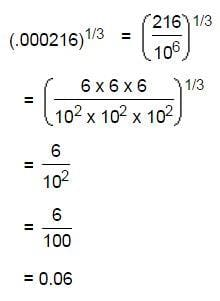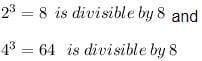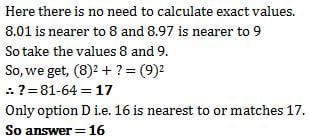# Test: Cubes

## 10 Questions MCQ Test Logical Reasoning (LR) and Data Interpretation (DI) | Test: Cubes

Description
Attempt Test: Cubes | 10 questions in 10 minutes | Mock test for UPSC preparation | Free important questions MCQ to study Logical Reasoning (LR) and Data Interpretation (DI) for UPSC Exam | Download free PDF with solutions
QUESTION: 1

### The cube root of .000216 is:

Solution:QUESTION: 2

### Cube root of 0.064 is

Solution:

0.064 = 64/1000= 4^3 / 10^3 =(4/10)^3= (2/5)^3

taking cube root of 0.064= 2/5=0.4

QUESTION: 3

### The cube of any multiple of 2 is always divisible by

Solution:

Cube of multiple of 2 is divisible by 8
eg:QUESTION: 4

What is the smallest number by which 3600 be divided to make it a perfect cube?

Solution:

Prime factorise 3600.

You will get 3600 = 2 x 2 x 2 x 2 x 3 x 3 x 5 x 5

Now group the numbers into groups of 3.

You will get 1 group of 2 and remaining numbers i.e. 2,3,3,5,5

Now multiply these extra numbers

You will get 2 x 3 x 3 x 5 x 5 = 450

So, 3600 divided by 450 = perfect cube (8)

QUESTION: 5

Cube of all odd natural numbers is

Solution:

Odd, Any odd number to power 3 will have unit digit odd

Ex.  7^3=3. 49^3=9.

QUESTION: 6

(8.01)2 + ? = (8.97)2 What will approximately come in place of question mark?

Solution:QUESTION: 7

The smallest number by which 5400 must be multiplied so that it become a perfect cube, is

Solution:

Find prime factors of 5400

5400=2×3×3×3×2×2×5×5

If we group them in the group of 3

5400=(2×2×2)×(3×3×3)×5×5

Here to make group of 3 for 5

We have to multiply 5400 by 5.

QUESTION: 8

Cube of all even natural numbers is

Solution:
QUESTION: 9

Cube of a natural number will never end with the digit

Solution:

There'd no such digit which can't end in a cube of a natural number. Hence a cube of a natural number can end with any digit.
1³= 1 (ends with 1)
2³= 8 (ends with 8)
3³= 27 (ends with 7)
4³= 64 (ends with 4)
5³= 125 (ends with 5)
6³= 216 (ends with 6)
7³= 343 (ends with 3)
8³= 512 (ends with 2)
9³= 729 (ends with 9)
0³= 0 (ends with 0)
Hence the last digits are 0,1,8,7,4,5,6,3,2,9
Therefore we see every digits are last digits of these cubes. Hence a cube of a natural number can end with any digit.

QUESTION: 10

Cube of 0.2 is

Solution:

0.2×0.2×0.2=0.008Use Code STAYHOME200 and get INR 200 additional OFF Use Coupon Code